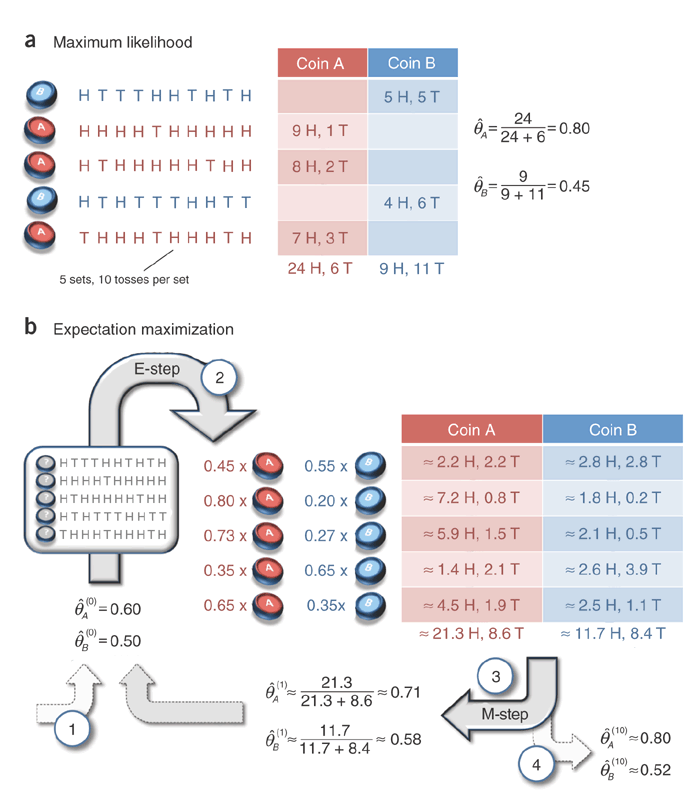keywords: Algorithm CJKmainfont: KaiTi –-

# EM算法

## 最大似然

$\begin{equation} \hat {\theta_A} = \frac {选择硬币A时正面朝上的次数} {选择硬币A的次数} \end{equation}$

## EMimport numpy as np
from scipy.stats import binom

observed = """
HTTTHHTHTH
HHHHTHHHHH
HTHHHHHTHH
HTHTTTHHTT
THHHTHHHTH"""

x = np.array([[1 if c=="H" else 0 for c in line] for line in observed.strip().split()])
x_tails = 10 - x.sum(axis=1)

def calc_z(theta):
return za_est / (za_est + zb_est), zb_est / (za_est + zb_est)

def calc_theta(z):
t_A = (x_tails * z).sum()
t_B = (x_tails * z).sum()
return h_A / (h_A + t_A), h_B / (h_B + t_B)

def is_nearly_same(theta_pre, theta_cur):
return np.abs(sum(theta_pre) - sum(theta_cur)) < 1e-5

theta_pre = [0.6, 0.5]

for i in range(1000):
theta_cur = calc_theta(calc_z(theta_pre))
if is_nearly_same(theta_pre, theta_cur):
print i, theta_cur
break
else:
theta_pre = theta_cur
# 11 (0.7967829009034072, 0.51959543422720311)